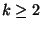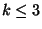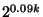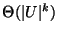Next: MINIMUM LENGTH EQUIVALENT FREGE Up: Propositional Logic Previous: MINIMUM EQUIVALENCE DELETION   Index

### MAXIMUM K-CONSTRAINT SATISFACTION

• INSTANCE: Set U of variables, collection C of conjunctive clauses of at most k literals, where a literal is a variable or a negated variable in U, and k is a constant,.

• SOLUTION: A truth assignment for U.

• MEASURE: Number of clauses satisfied by the truth assignment.

• Good News: Approximable within.

• Comment: Transformation from MAXIMUM 2-SATISFIABILITY. Approximable within 1.165 but not within 1.111 for k=2, and approximable within 2 but not within 2for k=3. There are also results of specific variations of the problem for. Not approximable withinfor large enough k . Not in APX when. When there are exactly k variables in each clause and the number of clauses isthe problem admits a randomized PTAS . A complete classification of the approximability of optimization problems derived from Boolean constraint satisfaction is contained in  (maximization) and in  (minimization).